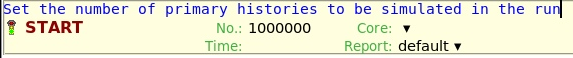# Two questions related BEAMAXES and Dose unit

Dear Fluka expert,

1. According to this post:

I defined my beam direction as you can see below:

But the results are strange. Did I make a mistake?

1. The Dose unit is GeV/g/primary. If I want to convert it to the eV/atom I should multiply it by primary. And The BEAMPART unit is particle/primary/cm^2.
If I understand correctly, according to the images below, the number of particles is 1e6, and the number of primaries is 5e6?Absorbed Dose.flair (2.4 KB)
Absorbed Dose.inp (1.7 KB)

I really appreciate your kind help.
Best regards,
Marziyeh

Hello Marziyeh,

The number of histories in the START card and the cumulated number of histories in the 5 cyclers you performed must not be confounded with the number of primaries. As the name already indicates, the number of histories indicates the number of possible “stories” of a primary particle with propoerties described by BEAM, BEAMAXES and a possible SOURCE-routine that were simulated by FLUKA in a “statistical experiment”. The outcome, for example the absorbed dose, is the mean value of all those experiments. Dividing the total number of “statistical histories” over several runs allows also to determine the first momentum of the probability distribution function of the results.

FLUKA results are given per primary particle, in your case per photon. To relate to an experiment, you have to multiply with the number of photons emitted in the experiment. Usually this number must be estimated, for example from the power: (energy per photon) * (photons per time unit) or other quantities which are accessible to measurement.
Greetings, Thomas ( @totto )

Dear @totto ,

I appreciate your help so much.

So I need to define pulse energy (pulse energy[joule] = number of photons * photon energy[eV]) or time or as you said source power. With which cards can I define these parameters?

And what about fluence [particle/primary/cm^2? If we consider the number of photons as the “primary”, what is the particle in this case?

Hi Marziyeh,

you simulate essentially the (mean) result for a single photon with FLUKA, all the rest you must calculate outside of the simulation program, based on independent information you have.

In your case, the source term eq 5 for the heat transport eq 4 contains the fluence I_0 and the pulse duration tau. Together with the cross section of the photon “beam” you can calculate the number of photons hitting the mirror, to be multiplied with the absorbed dose (or the fraction of absorbed dose by depth AEF(z)).
@totto

2 Likes

Dear Marzieyh,

I had a quick look at your input file, and my advice to you is to check first beam particle fluence (USRBIN - PHOTON or BEAMPART), instead of directly score the dose. This way you can quickly figure out if your beam shape (annular here) and beam start coordinates and the direction is as you want to simulate. In your input, you start your beam such that half of your annular shape beam is outside the Si layer.
I hope this helps.

Best regards,
Elzbieta

1 Like

Dear @totto ,